## Effect of Negative Feedback on Output Impedance:

Just as high input impedance is advantageous to an amplifier, so is low output impedance. With lower output impedance, the amplifier is better suited to drive a low impedance load. Such a desirable characteristic can be had by employing negative feedback. The Effect of Negative Feedback on Output Impedance of an amplifier is explained below.

### Voltage-Series Feedback: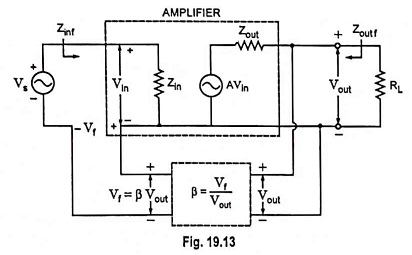The voltage-series feedback circuit given in Fig. 19.13 provides sufficient circuit detail for determining output impedance with feedback. The input terminals are short circuited so that – Vf is now the only input voltage to the amplifier. Now a voltage source Vout is applied at the output terminals so that lout current is drawn from the applied source.

Now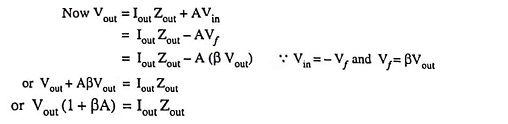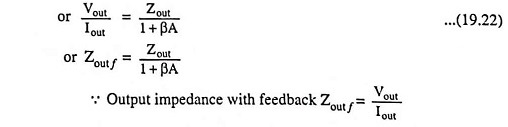Thus, series voltage negative feedback reduces the output impedance of an amplifier by a factor (1 + βA). This is the same factor by which voltage gain is reduced.

### Current-Shunt Feedback:

The output impedance with current shunt feedback can be determined by disconnecting the load resistance RL, applying a signal V to the output terminals with current Is removed. Now output current I is measured. The ratio of V to I provides the output impedance of circuit [Fig. 19.10 (d)].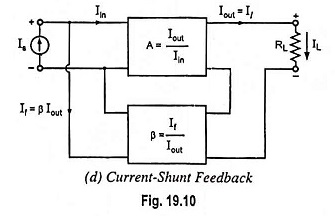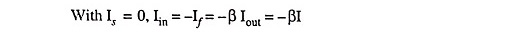Since current in the output circuit due to feedback opposes the current I, due to applied voltage, V

So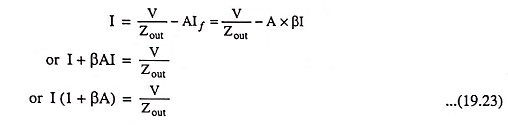and output impedance,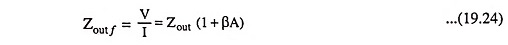So, output impedance is increased by the factor (1 + βA).

### Current-Series Feedback: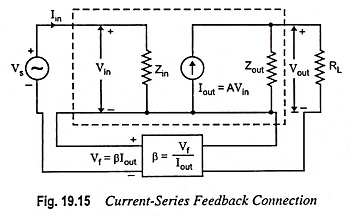The output impedance with current-series feedback can be determined by disconnecting load resistance RL, applying a signal V to the output with Vs shorted out. The ratio of V to I provides the output impedance of the circuit. Now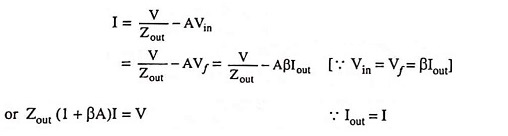or Output impedance,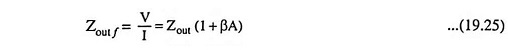So, the output impedance is increased by the factor (1 + βA).

Scroll to Top